• 图像增强处理：设计一套空间域与频率域结合的图像增强算法，处理以下任一组图片中的带噪声图像，去除噪声，提高图像质量。 （1）已知：噪声为随机噪声和周期噪声混合噪声； （2）要求： a）去噪处理后，计算均方误差...
• 这是能够实现图像频率域增强的C++代码 在频率域中实现增强图像的效果
• 通过MATLAB进行频率域图像增强实验，注意文件和图片在同一目录下
• 遥感图像频率域增强,详细介绍几种图像频率域增强的方法
•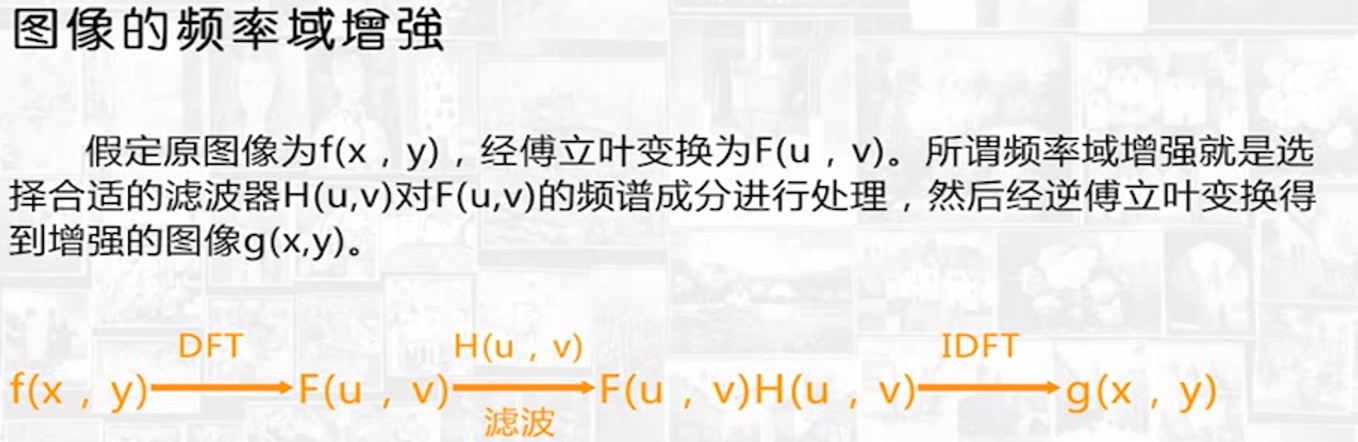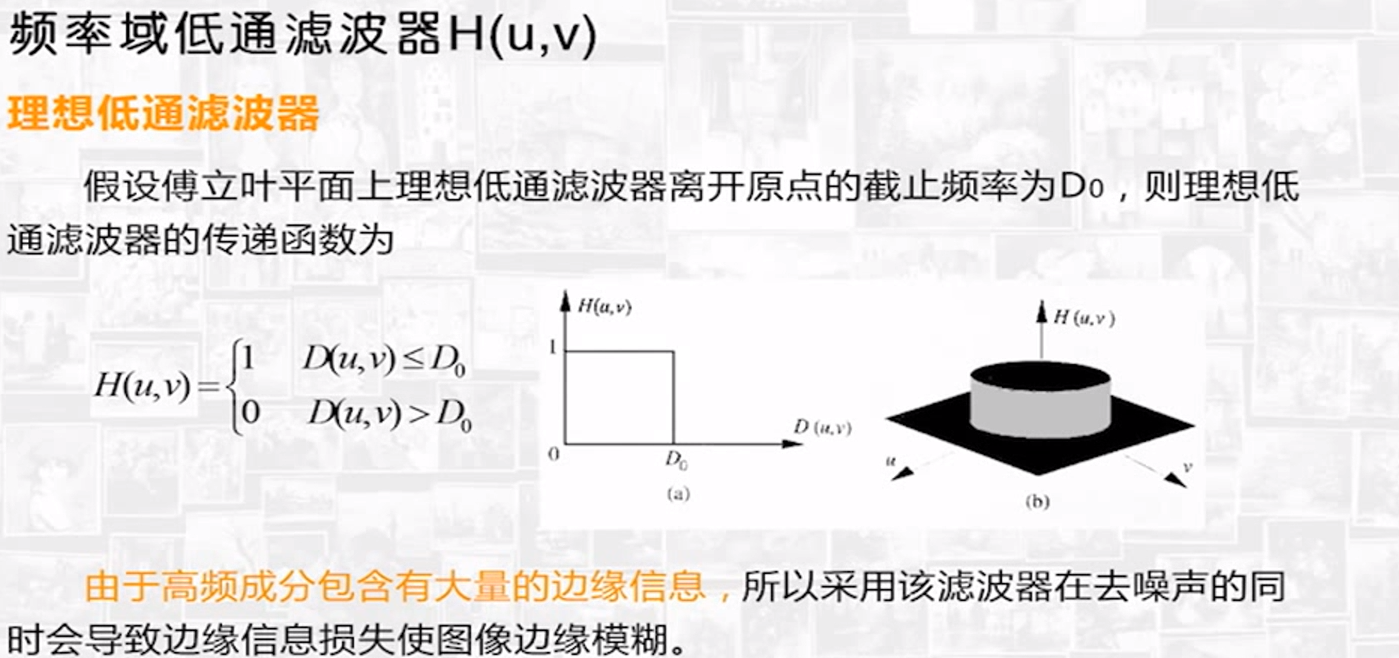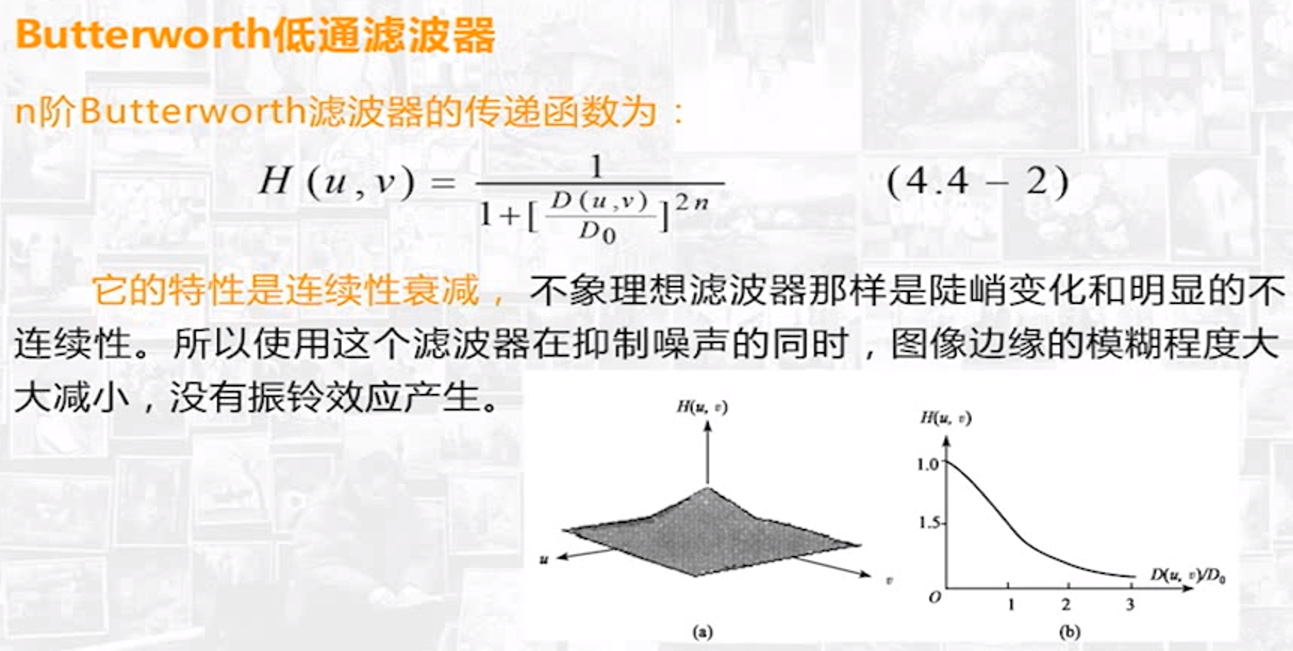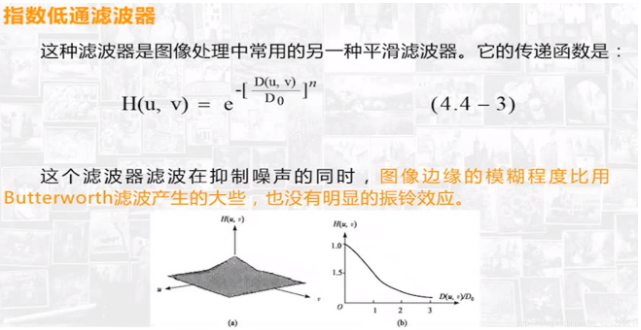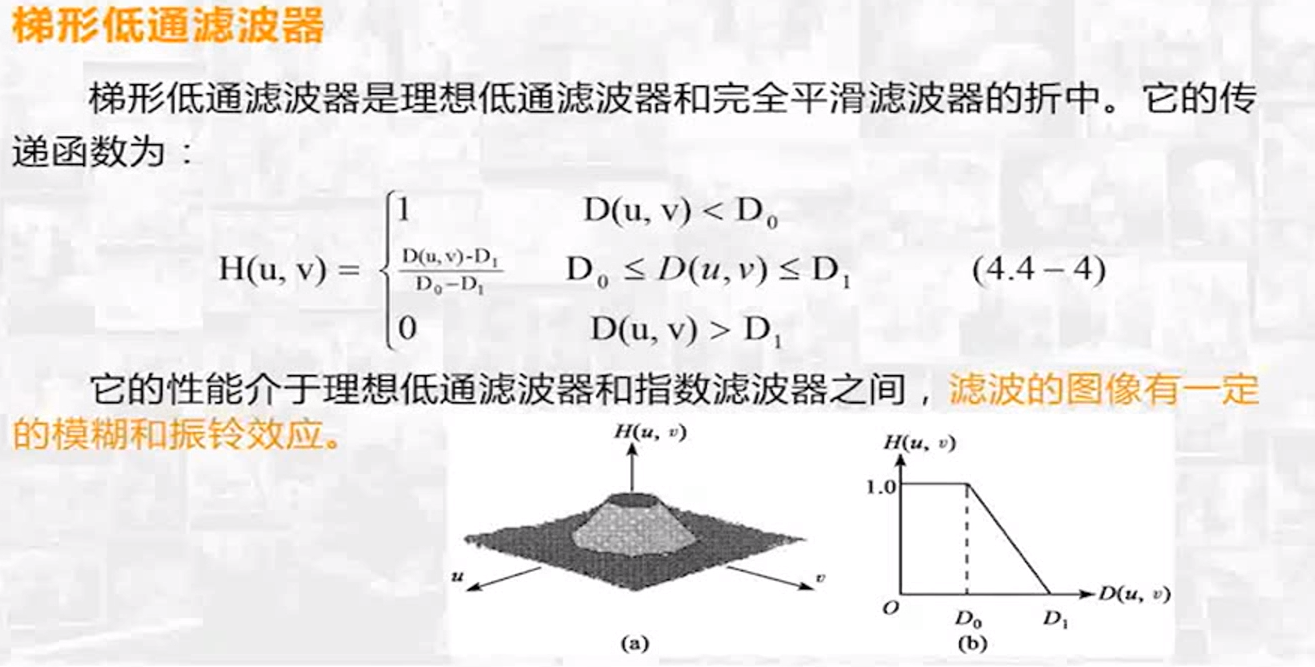展开全文• ## 频率域图像增强

千次阅读 2018-08-03 17:59:26
空间域图像增强频率域图像增强是两种截然不同的技术，实际上在相当程度上说它们是在不同的领域做相同的事情，是殊途同归的，，只是有些滤波更适合在空间域完成，而有些则更适合在频率域中完成   2傅里叶变换...
1频率域滤波与空间域滤波殊途同归
空间域图像增强与频率域图像增强是两种截然不同的技术，实际上在相当程度上说它们是在不同的领域做相同的事情，是殊途同归的，，只是有些滤波更适合在空间域完成，而有些则更适合在频率域中完成

2傅里叶变换基础知识
傅里叶级数：法国数学家傅里叶发现任何周期函数只要满足一定条件（狄利赫里条件），都可以用正弦函数和余弦函数构成无穷级数，却以不同频率的正弦和余弦函数的加权来表示，称为傅里叶级数。
傅里叶变换的实质——基的转换：对于给定函数f(x)，关键是选择合适的基，使得f(x)在这组基下，表现出需要的特性。当某一组基不满足要求时，就需要通过变换函数转换到另一组基下

3快速傅里叶变换及其实现
fft2（）函数：该函数用于执行二维快速傅里叶操作，因此可以直接用于图像处理
Y=fft2（X）
Y=fft2(X,m,n)
X为输入图像，m和n分别用于将X的第一和第二维规整到指定的长度，当m和n均为2 的整数次幂时算法的执行速度要比m和n为素数时更快。
Y是计算得到的傅里叶频谱，是一个复数矩阵
注意：计算abs（Y）可以得到幅度谱，计算angle（Y）可以得到相位谱
fftshift（）函数：利用了频谱的周期性特点，将输出图像的一半平移到另一端，从而使零频被移动到图像的中间。
Y=fftshift（X）
Y=fftshift（X，dim）
X为要平移的频谱，dim指出了在多维数组的哪个角度上执行平移操作。Y是经过平移的频谱
ifft2（）函数：该函数用于对图像（矩阵）执行逆傅里叶变换。输出矩阵的大小与输入矩阵相同。
Y=ifft2（X）
Y=ifft2（X，m，n）
X为要计算反变换的频谱；m，n的意义与fft2（）中相同；Y是反变换后得到的原始图像
matlab实现（幅度谱的意义示例）
subplot(1,2,1); imshow(temp,[]); title('FFF'); subplot(1,2,2); imshow(I1); title('Source');
fcoef=fft2(I2);%做fft变换 spectrum=fftshift(fcoef);%将零点移到中心 temp=log(1+abs(spectrum));%对幅值做对数变换以压缩动态范围
figure; subplot(1,2,1); imshow(temp,[]); title('FFF'); subplot(1,2,2); imshow(I2); title('Source');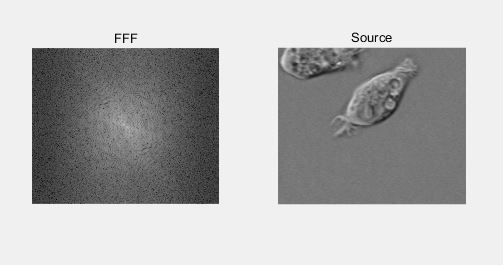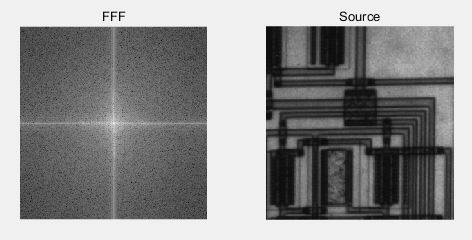美女与猫——交换两幅图像的相位谱
%求傅里叶变换 Af=fft2(A); Bf=fft2(B);
%分别幅度谱和相位谱 AfA=abs(Af); AfB=angle(Af);
BfA=abs(Bf); BfB=angle(Bf);
%交换相位谱并重建复数矩阵 AfR=AfA .*cos(BfB)+AfA .*sin(BfB) .*i; BfR=BfA .*cos(AfB)+BfA .*sin(AfB) .*i;
%傅里叶反变换 AR=abs(ifft2(AfR)); BR=abs(ifft2(BfR));
%显示图像 subplot(2,2,1); imshow(A); title('美女原图像');
subplot(2,2,2); imshow(B); title('猫的原图像');
subplot(2,2,3); imshow(AR,[]); title('美女的幅度谱与猫的相位谱组合');
subplot(2,2,4); imshow(BR,[]); title('猫的幅度谱与美女的相位谱组合');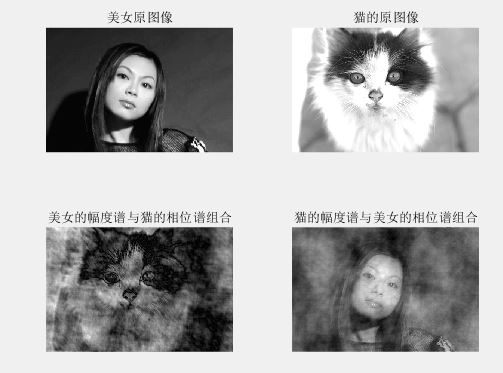4频域滤波基础
频域滤波与空间域滤波的关系：傅里叶变换可以将图像从空域变换到频域，而傅里叶反变换则可以将图像的频谱逆变换为空域图像，也即人可以直接识别的图像。
注意：将频谱原点移至图像中心，因此需要构造对应的原点在中心的滤波器，并在滤波之后使用iffshift（）函数将原点移回以进行反变换

5频率域低通滤波器
理想低通滤波器：最容易想到的衰减高频成分的方法就是在一个称为“截止频率”的位置“截断”所有的高频成分，将图像频谱中所有高于这一截止频率的频谱成分设为0，低于截止频率的成分保持不变。
高斯低通滤波器：
%生成滤镜 ff=imgaussflpf(I,20); %应用滤镜 out=imfreqfilt(I,ff);
figure(1); subplot(2,2,1); imshow(I); title('Source');
%计算FFT并显示 temp=fft2(I); temp=fftshift(temp); temp=log(1+abs(temp)); figure(2); subplot(2,2,1); imshow(temp,[]); title('Source');
figure(1); subplot(2,2,2); imshow(out); title('Gauss LPF,sigma=20');
%计算FFT并显示 temp=fft2(out); temp=fftshift(temp); temp=log(1+abs(temp)); figure(2); subplot(2,2,2); imshow(temp,[]); title('Gauss LPF,sigma=20');
%生成滤镜 ff=imgaussflpf(I,40); %应用滤镜 out=imfreqfilt(I,ff);
figure(1); subplot(2,2,3); imshow(out); title('Gauss LPF,sigma=40');
%计算FFT并显示 temp=fft2(out); temp=fftshift(temp); temp=log(1+abs(temp)); figure(2); subplot(2,2,3); imshow(temp,[]); title('Gauss LPF,sigma=40');
%生成滤镜 ff=imgaussflpf(I,60); %应用滤镜 out=imfreqfilt(I,ff);
figure(1); subplot(2,2,4); imshow(out); title('Gauss LPF,sigma=60');
%计算并显示 temp=fft2(out); temp=fftshift(temp); temp=log(1+abs(temp)); figure(2); subplot(2,2,4); imshow(temp,[]); title('Gauss LPF,sigma=60');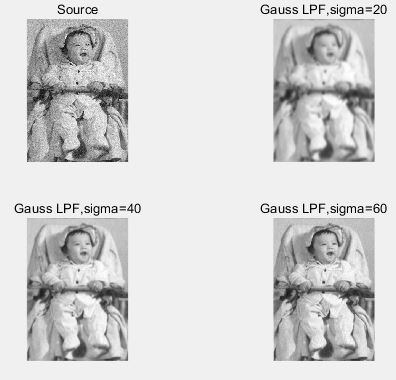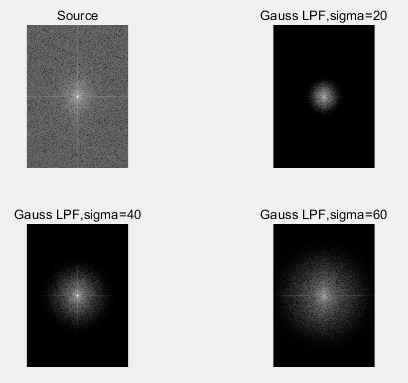6频率域高通滤波器：图像锐化可以通过衰减图像频谱中的低频成分来实现，这就建立了空间域图像锐化与频域高通滤波器之间的对应关系。
matlab实现
%生成滤镜 ff=imgaussfhpf(I,20); %应用滤镜 out=imfreqfilt(I,ff);
figure(1); subplot(2,2,1); imshow(I); title('Source');
%计算FFT并显示 temp=fft2(I); temp=fftshift(temp); temp=log(1+abs(temp)); figure(2); subplot(2,2,1); imshow(temp,[]); title('Source');
figure(1); subplot(2,2,2); imshow(out); title('Gauss HPF,sigma=20');
%计算FFT并显示 temp=fft2(I); temp=fftshift(temp); temp=log(1+abs(temp)); figure(2); subplot(2,2,2); imshow(temp,[]); title('Gauss HPF,sigma=20');
%生成滤镜 ff=imgaussfhpf(I,40); %应用滤镜 out=imfreqfilt(I,ff);
figure(1); subplot(2,2,3); imshow(out); title('Gauss HPF,sigma=40');
%计算FFT并显示 temp=fft2(I); temp=fftshift(temp); temp=log(1+abs(temp)); figure(2); subplot(2,2,3); imshow(temp,[]); title('Gauss HPF,sigma=40');
%生成滤镜 ff=imgaussfhpf(I,60); %应用滤镜 out=imfreqfilt(I,ff);
figure(1); subplot(2,2,4); imshow(out); title('Gauss HPF,sigma=60');
%计算FFT并显示 temp=fft2(I); temp=fftshift(temp); temp=log(1+abs(temp)); figure(2); subplot(2,2,4); imshow(temp,[]); title('Gauss HPF,sigma=60');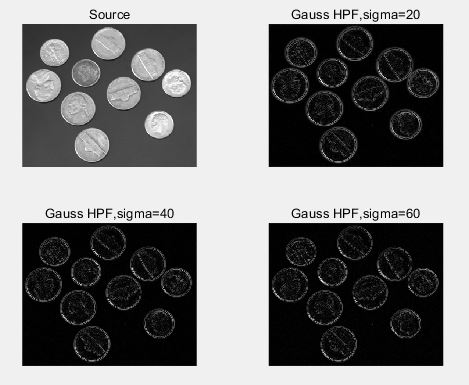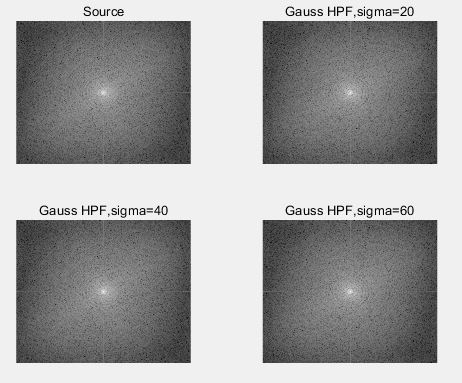高斯高通滤波器可以较好的提取图像中的边缘信息，sigma取值越小，边缘提取越不精确，会包含越多的非边缘信息；sigma取值越大，边缘提取越精确，但可能包含不完整的边缘信息。
频域拉普拉斯滤波器

ff=imlapf(I); out=imfreqfilt(I,ff);
figure(1); subplot(1,2,1); imshow(I); title('Source');
temp=fft2(I); temp=fftshift(temp); temp=log(1+abs(temp)); figure(2); subplot(1,2,1); imshow(temp,[]); title('Source');
figure(1); subplot(1,2,2); imshow(out); title('Laplace Filter');
temp=fft2(out); temp=fftshift(temp) temp=log(1+abs(temp)); figure(2); subplot(1,2,2); imshow(temp,[]); title('Laplace Filter');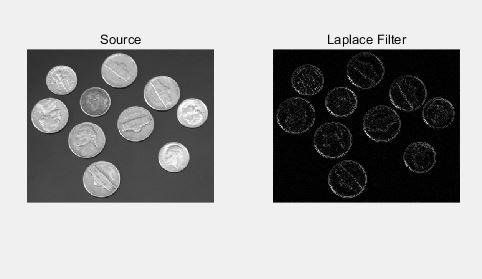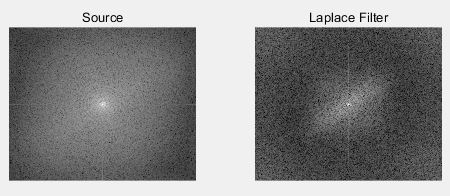7利用频域滤波消除周期噪声
频域带阻滤波器：阻止频谱中某一频带范围的分量通过，其他频率成分则不受影响。
添加周期性噪声前后对比图：
O=imread('pout.tif'); [M,N]=size(O); I=O; for i=1:M; for j=1:N;     I(i,j)=I(i,j)+20*sin(20*i)+20*sin(20*j);%添加周期噪声 end end
subplot(1,2,1); imshow(O); title('Source');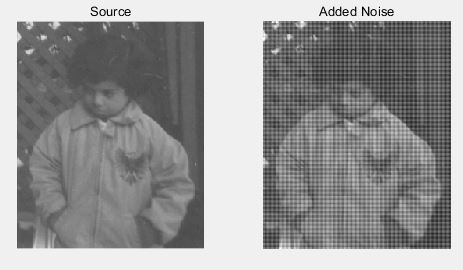频谱分析：
i_f=fft2(I); i_f=fftshift(i_f); i_f=abs(i_f); i_f=log(1+i_f);
o_f=fft2(O); o_f=fftshift(o_f); o_f=abs(o_f); o_f=log(1+o_f);
figure(1); imshow(o_f,[]); title('Source');8频率域滤波与空间域滤波之间的内在联系
频域滤波较空域滤波而言更为直观，频域下滤波器表达了一系列空域（平滑、锐化等）的本质，即对高于/低于某一特定频率的灰度变化信息予以滤除，而对其他的灰度变化信息基本保持不变。这种直观性增加了频域滤波器设计的合理性。

补充:高频与低频的区别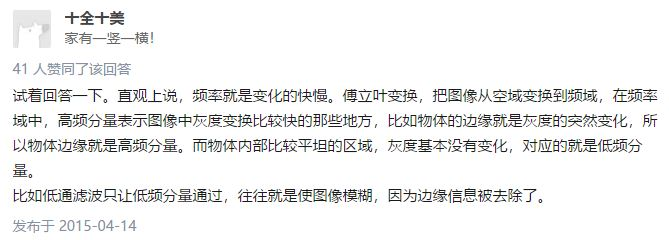展开全文• 频率域图像增强 空间域和频率域为我们提供了不同的视角。在空间域中，函数的自变量 (x,y) 被视为二维空间中的一点，数字图像 f(x,y) 即为一个定义在二维空间中的矩形区域上的离散函数；换一个角度，如果将 f(x,y) 视...
频率域图像增强
空间域和频率域为我们提供了不同的视角。在空间域中，函数的自变量 (x,y) 被视为二维空间中的一点，数字图像 f(x,y) 即为一个定义在二维空间中的矩形区域上的离散函数；换一个角度，如果将 f(x,y) 视为幅值变化的二维信号，则可以通过某些变换手段（如傅里叶变换、离散余弦变换、沃尔什变换和小波变换等）在频率域下对它进行分析。
1、频率域滤波——与空间域滤波殊途同归
在很多情况下，频率域滤波和空间域滤波可以视为对同一个图像增强问题的殊途同归的两种解决方法。而在另外一些情况下，有些增强问题更适合在频率域中完成，有些则更适合在空间域中完成。
傅里叶变换提供了一种变换到频率域的手段，由于用傅里叶变化表示的函数特征可以完全通过傅里叶反变换进行重建，不丢失任何信息，因此它可以使我们工作在频率域，而在转换回空间域时不丢失任何信息。

2、傅里叶变换基础知识
2.1、傅里叶级数
法国数学家傅里叶发现任何周期函数只要满足一定条件（狄利赫里条件）都可以用正弦函数和余弦函数构成的无穷级数，即以不同频率的正弦和余弦函数的加权和来表示，后世称为傅里叶级数。
对于有限定义域的非周期函数，可以对其进行周期延拓，从而使其在整个扩展定义域上为周期函数，从而也可以展开为傅里叶级数。

傅里叶级数的三角形式 周期为 T 的函数 f(t) 的三角形式傅里叶级数展开为࿱

展开全文图像处理
• 频率域图像增强：以修改图像的傅里叶变换为基础的方法； 高、低通滤波，同态滤波。 另外还有彩色图像的增强。 1.空间域图像增强 1.1基于灰度变换的图像增强 属于点处理技术，这部分内容参考之前的笔记点运算部分...
图像增强Image Enhancement：采用某种技术手段，改善图像的视觉效果，获奖图像转换成更适合人眼观察和机器分析、识别的形式，以便从图像中获取更有用的信息。1
空间域图像增强：针对图像自身，以对图像的像素直接处理为基础的方法； 点处理，模板处理；频率域图像增强：以修改图像的傅里叶变换为基础的方法； 高、低通滤波，同态滤波。
另外还有彩色图像的增强。
1.空间域图像增强
1.1基于灰度变换的图像增强
属于点处理技术，这部分内容参考之前的笔记点运算部分内容 ,有线性变换、幂次变换等。
1.2基于直方图处理的图像增强
灰度级直方图统计了不同灰度级出现的概率。
I=imread('marketspace.jpg');
I=rgb2gray(I);
% I=double(uint8(I));
for i=1:256
h(i)=sum(sum(I==i-1));
end
subplot(1,2,1);imshow(I);
subplot(1,2,2);plot(h);直方图均衡化
直方图均衡化处理，使得图像的灰度分布趋向均匀，图像所占有的像素灰度间距拉开，进而加大图像反差，达到增强目的。适用于一些由于灰度分布集中在较窄区间内的图像。
I=imread('marketspace.jpg');
I=rgb2gray(I);
K=32;
H=histeq(I,K);
figure;
subplot(2,2,1);imshow(I);
subplot(2,2,2);imshow(H);hold on;
subplot(2,2,3),hist(double(I),16),
subplot(2,2,4),hist(double(H),16),

结果：分析： 变换后直方图趋于平缓，灰级减少，灰度合并；原图像含像素多的几个灰级间隔被拉大，压缩的只是像素少的几个灰度级，实际视觉能接收的信息量增强了。可以看到增强的效果还是不错的，图中云层部分的细节有所增强。
1.3空间域滤波增强
属于模板处理技术，对图像进行滤波以去除图像噪声或增强图像细节。
空间域平滑滤波器
图像平滑（去噪）：抑制噪声、改善图像质量，通过积分使图像边缘模糊。
I=rgb2gray(imread('11.png'));
figure;
subplot(1,5,1),imshow(I),title("原始图像");
N=imnoise(I, 'salt & pepper', 0.06);
subplot(1,5,2),imshow(N),title("加椒盐噪声后的图像");
% 1）均值滤波
S=imfilter(N,fspecial('average', 5));
subplot(1,5,3),imshow(S),title("5*5领域平滑");
% 2）超限像素平滑法
T=20;
[m,n]=size(I);
C=zeros(m,n);
for i=1:m
for j=1:n
if abs(I(i, j)-S(i,j))>T
C(i,j)=S(i,j);                      % if |I(x,y)-S(x,y)|>T then G(x,y)=S(x,y)
else
C(i,j)=I(i,j);                       % if |I(x,y)-S(x,y)|<=T then G(x,y)=I(x,y)
end
end
end
subplot(1,5,4),imshow(uint8(C));title("超限像素平滑法");
% 3）高斯平滑线性滤波法
G=imfilter(N, fspecial('gaussian', [3 3], 0.9), 'replicate');
subplot(1,5,5),imshow(G),title("高斯平滑滤波");

结果：分析： （1）超限像素平滑是对局部平滑法的一种改进，对抑制椒盐噪声比较有效，对保护仅有微小灰度差的细节及纹理也有效。 随着邻域增大，去噪能力增强，但模糊程度也增大。 （2）高斯滤波器，对于高斯噪声的去噪效果较好。
空间域锐化滤波器
图像锐化：增强图像的边缘和轮廓，通过微分使图像边缘清晰。
I=rgb2gray(imread('11.png'));
figure;
subplot(1,5,1),imshow(I),title("原始图像");
N=imnoise(I, 'salt & pepper', 0.02);
subplot(1,5,2),imshow(N),title("加椒盐噪声后的图像");
S=imfilter(N, fspecial('sobel'));
subplot(1,5,3), imshow(S),title("Sobel滤波");
L=imfilter(N, fspecial('laplacian', 0.2));
subplot(1,5,4),imshow(L),title("拉普拉斯滤波");
G=imfilter(N, fspecial('log', [5 5], 0.5));
subplot(1,5,5),imshow(G),title("高斯-拉普拉斯滤波");

结果：2.频率域图像增强
频率域处理工作主要是：

g

(

x

,

y

)

=

f

(

x

,

y

)

∗

h

(

x

,

y

)

\ g(x,y)=f(x,y)*h(x,y)\,

2.1频率域平滑滤波器
I=rgb2gray(imread('11.png'));
figure;
subplot(2,2,1),imshow(I),title("原始图像");
N=imnoise(I, 'salt & pepper', 0.02);
subplot(2,2,2),imshow(N),title("加椒盐噪声后的图像");
NF=fftshift(fft2(N));
[m,n]=size(N);
H=zeros(m,n);
% 1)理想低通滤波
T=105;
for i=1:m
for j=1:n
if sqrt((i-m/2)^2+(j-n/2)^2)<T
H(i,j)=1;
end
end
end
L=NF.*H;
L=ifft2(ifftshift(L));
subplot(2,2,3),imshow(uint8(real(L))),title("理想低通滤波");
% 2）巴特沃斯低通滤波器
T=105;
N=2;
for i=1:m
for j=1:n
d=sqrt((i-m/2)^2+(j-n/2)^2);
h=1/(1+(d/T)^(2*N));
H(i,j)=h*NF(i,j);
end
end
B=ifft2(ifftshift(H));
subplot(2,2,4),imshow(uint8(real(B))),title("巴特沃斯滤波");

结果（为了显示两种滤波器的效果区别，对图像进行了局部放大）：分析： （1）理想低通滤波器会产生振铃现象使图像变得模糊，相对而言，巴特沃斯滤波器没有陡峭的变化，图像边缘的模糊程度减小。 （2）阈值T越大，图像的模糊程度越低。
2.2频率域锐化滤波器
程序和上一个程序类似，只是公式稍有调整。
% 1)理想高通滤波
T=35;
for i=1:m
for j=1:n
if sqrt((i-m/2)^2+(j-n/2)^2)>T
H(i,j)=1;
end
end
end
L=NF.*H;
L=ifft2(ifftshift(L));
subplot(2,2,3),imshow(uint8(real(L))),title("理想低通滤波");
% 2）巴特沃斯高通滤波器
T=35;
N=2;
for i=1:m
for j=1:n
d=sqrt((i-m/2)^2+(j-n/2)^2);
h=1/(1+(T/d)^(2*N));
H(i,j)=h*NF(i,j);
end
end
B=ifft2(ifftshift(H));
subplot(2,2,4),imshow(uint8(real(B))),title("巴特沃斯滤波");

结果：分析： （1）巴特沃斯高通滤波器的效果较于理想高通滤波器好一点； （2）阈值T的值越小，锐化程度越高。
2.3同态滤波器
同态滤波把频率滤波和灰度变换结合：
首先对非线性混杂信号做对数运算，把两个相乘的分量变为两个相加的分量，它们分别代表图像的高频和低频分量；然后用线性滤波方法处理；最后做逆运算，恢复处理后图像。
可以应用于消除光照不足带来的影响，同时又不损失图像细节。
I0=rgb2gray(imread('astronaut.jpg'));
I1=log(double(I0)+1);
I2=fft2(I1);
[m,n]=size(I2);
N=2;c=2;
T=7;
rh=2;rl=0.5;
for i=1:m
for j=1:n
d=sqrt(i^2+j^2);
h=rl+(rh-rl)*(1/(1+(T/(c*d))^(2*N)));
I2(i,j)=I2(i,j)*h;
end
end
I3=ifft2(I2);
I4=(exp(I3)-1);
figure,
subplot(1,2,1),imshow(I0);
subplot(1,2,2),imshow(uint8(real(I4)));

结果：分析： （其实觉得这个结果没有很好，而且实验了很多照片，也没有找到效果好的）结果就是增强了图片的对比度，而且，阈值T越大，曝光度越小。

出自杨杰主编的《数字图像处理及MATLAB实现（第2版）》 ↩︎

展开全文• 文章目录频率域图像增强1. 傅里叶变换1.1 一维傅里叶变换总结 频率域图像增强 1. 傅里叶变换 1.1 一维傅里叶变换 单变量连续函数f(x)的傅里叶变换F(u)定义为等式： 反变换可以定义为： 上述这两个式子，就反映了...计算机视觉
• 频域增强指在图像的频率域内，对图像的变换系数（频率成分）直接进行运算，然后通过Fourier逆变换以获得图像的增强效果。
• 1、在图像中，像元的灰度值随位置变化的频繁程度可以用频率来表示，这是一种随位置变化的空间频率。 ...是指连续像元的灰度值的最高值与最低值的差。... 滤波是指在图像空间域(x,y)或者频率域...
• dft2d函数为对灰度图进行离散傅里叶变换和反变换 filter2d_freq为对灰度图进行频率域滤波 修改Runner函数中的图片路径然后运行即可
• 图像在变换加移动中心后，从中间到外面，频率上依次是从低频到高频的， 那么我们如果把中间规定一小部分去掉，是不是相对于把低频信号去掉了呢？ 这也就是相当于进行了高通滤波 低通滤波器：把上述模板中的1...
•   本篇文章仅作为博主学习笔记使用，各位小伙伴可以简单浏览大概了解一下图像增强方面的知识呀????，可能不是特别全面呦~ Background   图像增强（Image Enhancement）是一种基本的图像处理技术，主要是为了改善...数字图像处理
• 总结最近项目中常用的图像增强方法： 一. 非锐化模板（钝化模板） 非锐化模板的处理步骤： 1. 高斯低通滤波图像 2. 从原图像减去模糊图像（产生的差值图像称为模板） 3. 将模板加到原图像上 二. 高提升滤波 ...
• 医学图像处理技术：ch4 频率域图像增强.ppt
• ## 频率域图像增强及MATLAB实现

万次阅读 多人点赞 2017-10-14 20:06:25
频域滤波和空间滤波可以视为对同一个图像增强问题的两种解决方式，二者殊途同归 。在一些情况下，图像增强问题适合在频域中完成，另一些情况下，适合在空间中完成，我们常常根据需要在二者之间做抉择，或是在...
• ## 频率域波图像增强

千次阅读 2016-12-27 20:32:27
频率域图像增强 高通滤波器和低通滤波器 本章的典型案例分析 利用频域滤波消除周期噪声 频域滤波基础频域滤波与空域滤波的关系 傅立叶变换可以将图像从空域变换到频域，而傅立叶反变换则可以将图像的频谱逆变换为...高通 图像处理
•图像变换
• 频率域滤波 时域(时间域):描述数学函数或物理信号与时间的关系，例如一 个信号的时域波形可以表达信号随着时间的变化。 频域(频率域):自变量是频率，即横轴是频率，纵轴是该频率信 号的幅度。也就是通常说的频谱图。...
• 棱镜是可以将光分解为不同颜色的物理仪器，每个成分的颜色由波长（或频率）来决定。傅里叶变换可以看作是数学上的棱镜，将函数基于频率分解为不同的成分。当我们考虑光时,讨论它的光谱或频率谱。同样, 傅立叶变换...
• 1.fft2()函数Y=fft2(X,m,n);...对频谱进行处理（如log压缩舒展处理），在把频谱转换成空间图像。Xf=ifft2(Ys);%傅里叶反变换有意思的交换相位谱% c6s2.m% 读取图片 A = rgb2gray(imresize(imread('G:\0ShiJue\matlab
• 循序渐进之（七）频率域图像增强之傅里叶变换梳理 一.基本知识 傅里叶变换是重要的一个方面，同时也是比较难缠的一个方面，这里在学习大佬关于傅里叶讲解的基础上，简单梳理，争取直白化，由于数学功底欠佳，...傅里叶变换 DFT 傅里叶级数 图像处理DFT
• 冈萨雷斯《MATLAB数字图像处理教程》第四章“频率域增强冈萨雷斯...